Binary Logic, Gates, and Boolean Algebra

Tom Kelliher, CS 240

Feb. 1, 2010

Introduction

Outline

1. Binary logic and gates.

2. Boolean Algebra.

Coming Up

Standard forms, maps, and minimization.

Binary Logic and Gates

1. Fundamental operators and their symbols:
1. AND

2. OR

3. NOT

2. NAND is complete.

3. Gate fan-in and fan-out. Electrical significance.

4. Timing diagram.
1. Frequency and period.

2. Timing diagrams. Show AND, OR, NOT waveforms for input: A: 0011, B: 0101.

3. What do the waveforms really look like: propagation delay, noise, under- and over-shoot.

Boolean Algebra

1. Boolean functions can be represented by equations, truth tables, or logic circuits.

How do you convert from one form to another?

How many rows in the truth table of an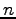-input Boolean function?

2. Why would we want to simplify a Boolean equation?

3. Basic Identities:
 1.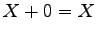2.3.4.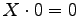5.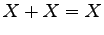6.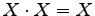7.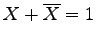8.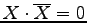9.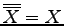10.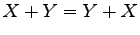11.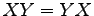12.13.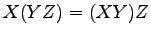14.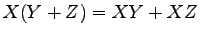15.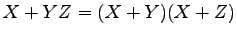16.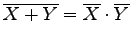17.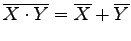Exercises

Example simplification. Use Boolean manipulation to show: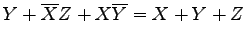Thomas P. Kelliher 2010-01-29
Tom Kelliher